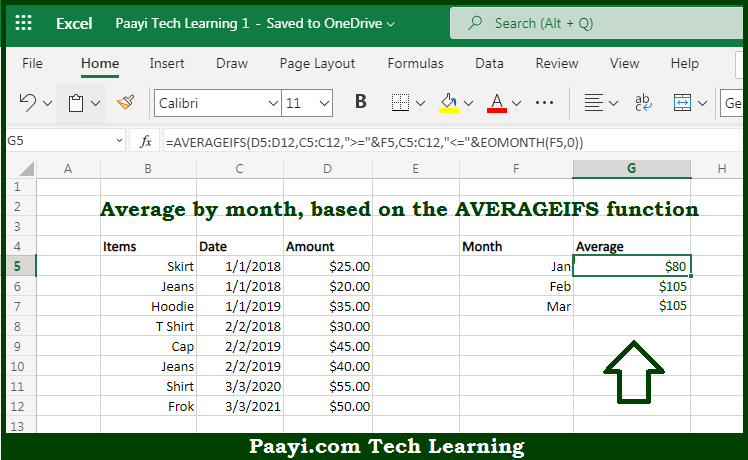# Learn How to AVERAGE By Month in Microsoft Excel

Written by | 0 Comments | 552 Views

In this article, you will learn how to SUM various things in Microsoft Excel using a single/combination(s) of functions. You will also know how to AVERAGE By Month and see the generic formula.

AVERAGE By Month in Microsoft Excel

The main purpose of this formula is to average by month. Here we will learn how to average the given date range by month in the workbook in Microsoft Excel. That implies, with the help of a formula based on the AVERAGEIFS and EOMONTH function you can able to average by month. So, with the help of this formula, you can able to average the given date range by month in the workbook in Microsoft Excel.

General Formula to AVERAGE By Month

=AVERAGEIFS(values,dates,">="&A1,dates,"<="&EOMONTH(A1))

The Explanation for the AVERAGE By MonthSo we know that with the help of the given formula above you can able to average by month. Here we will learn how to average the given date range by month in the workbook in Microsoft Excel. As we know that the AVERAGEIFS function is used to average ranges based on multiple criteria. In the example provided here, we are configuring the AVERAGEIFS  function to average amounts by month using these two criteria - first to match dates greater than or equal to the first day of the month. And second is to match dates less than or equal to the last day of the month.. So, with the help of this formula, you can able to average the given date range by month in the workbook in Microsoft Excel.